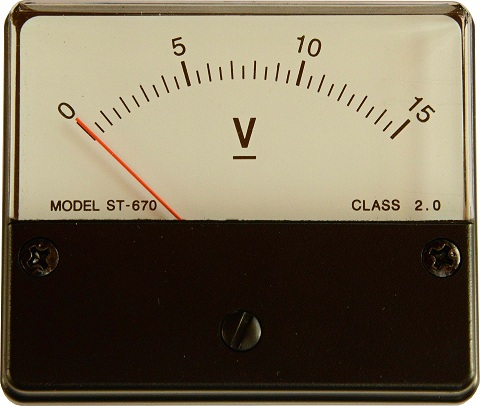# Difference Between Galvanometer and Voltmeter

## Main Difference – Galvanometer vs. Voltmeter

Galvanometers and voltmeters are measuring devices which are often used to evaluate electric circuits. The main difference between galvanometer and voltmeter is that a galvanometer is a type of device which has parts that move in response to an electric current, whereas a voltmeter is a device that is used to measure the potential difference (voltage) in a circuit. We will see that galvanometers can be used to measure not only voltages but also currents and that voltmeters can be constructed in different ways, with or without using a galvanometer.

## What is a Galvanometer

A galvanometer is a device that has a permanent magnet, inside which a coil of wire is placed. When a current passes through the coil of wire, the wire creates its own magnetic field. This magnetic field interacts with the magnetic field of the permanent magnets and, as a result, a force is exerted on the coil. The force causes the coil to move. A needle can be attached to the coil of wire and placed along a scale so that its motion can be measured. The larger the current through the coil, the larger is the force that the needle feels and therefore, the more it will move. This would cause the needle to deviate further from its resting position. If currents of known sizes are passed through the coil, the scale can be calibrated to read off any value of current within a given range. As a result, galvanometers can be used as ammeters to measure the size of an electric current. A galvanometer which functions in this way is called a moving coil galvanometer since it is the motion of the coil that causes the needle to move along the scale. It is also possible to construct galvanometers whose coils do not move, but the magnets do. These type of galvanometers are called moving magnet galvanometers.A moving coil galvanometer schematic (left) and a photograph of an old moving coil galvanometer (right)

Galvanometers are not used for measurements alone. The magnet of a moving magnet galvanometer can be attached to a mirror so that when the magnets move in response to electric currents in the coil, the mirror rotates along with the magnets. This means that the mirror’s movements can be controlled by controlling the current. This type of a setup is often referred to as mirror galvanometers. They are used to control beams of light in some types of projectors. They are also sometimes used to direct high-intensity laser beams onto materials for laser etching. For instance, the video below shows how a mirror galvanometer can be used to make engravings on wood using a laser:

## What is a Voltmeter

A voltmeter is a device which, when connected across two points on an electric circuit, measures the potential difference between those two points. Galvanometers can be used to make voltmeters. The needle of a galvanometer moves in response to current, but if we know the resistance of the coil, then we can use Ohm’s law to determine the corresponding potential difference between the two ends of a voltmeter. Once again, we could set up a scale next to the needle that reads the values of potential difference corresponding to the needle’s position.A moving-oil galvanometer being used as an analog voltmeter.

However, not all voltmeters are galvanometers. Traditionally, most voltmeters had been galvanometers. However today, digital voltmeters are used most often. These consist of an analog-to-digital converter (ADC) that converts any voltage that it receives into a corresponding digital value in the voltmeter’s circuit. The value is passed over to a display, where it is simply shown on a screen as a number.

## Difference Between Galvanometer and Voltmeter

### What it does

galvanometer is a device which moves in response to an electric current.

A voltmeter is a device that is used to measure a potential difference (voltage) in electric circuits.

### As a Measuring Device

galvanometer can be calibrated to measure current as well as voltage.

voltmeter only ever measures voltage.

### Other functions

Galvanometers are also used to control beams of light in response to a current.

Voltmeters are only used to measure voltage.

Image Courtesy:

“Diagram of D’Arsonval/Weston type galvanometer.” by Fred the Oyster (File:Galvanometer scheme.png) [CC BY-SA 3.0], via Wikipedia (Modified)

“A moving coil galvanometer.” by Wellcome Library, London (Library reference: Museum No. 116/1957, Photo number: M0016397) [CC BY 4.0], via Wikipedia (Modified)

“instrument-voltage-volt-meter-217276” by brudix (Own work) [CC0 1.0], via Pixabay